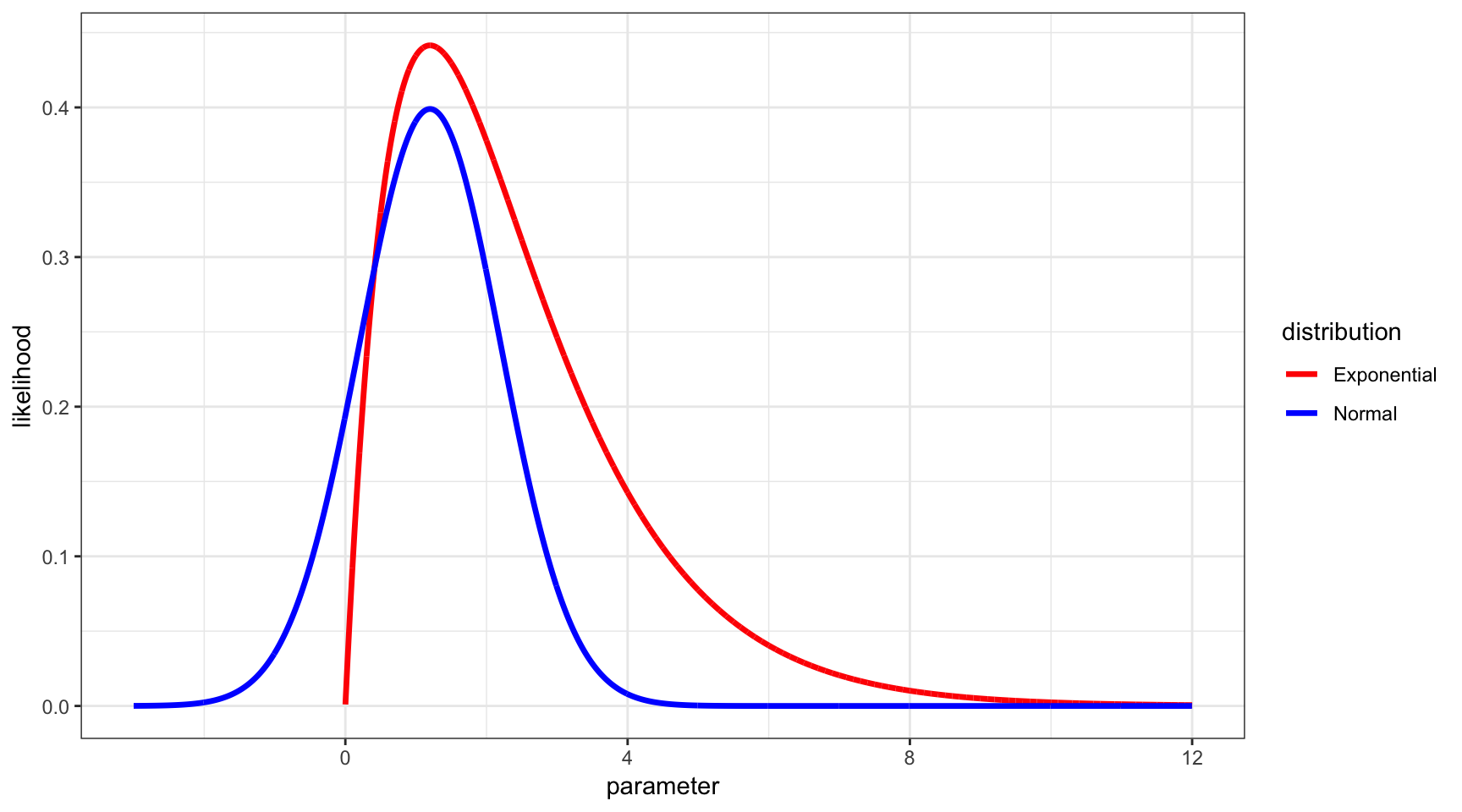32 Likelihood Function

32.1 Same MLE, Different $$L(\theta | \boldsymbol{x})$$32.2 Weighted Likelihood Estimate

Instead of employing estimator $$\hat{\theta}_{{\rm MLE}} = \operatorname{argmax}_\theta L(\theta ; \boldsymbol{x})$$, consider instead an arbitrary weight function, $$g(\theta)$$. We could take a weighted average of the likelihood function, assuming all of the integrals below exist.

$\tilde{\theta} = \frac{\int \theta g(\theta) L(\theta ; \boldsymbol{x}) d\theta}{\int g(\theta) L(\theta ; \boldsymbol{x}) d\theta}$

32.3 Conditional Expected Value

If we set

$h(\theta | \boldsymbol{x}) = \frac{g(\theta) L(\theta ; \boldsymbol{x})}{\int g(\theta^*) L(\theta^* ; \boldsymbol{x}) d\theta^*}$

then $$h(\theta | \boldsymbol{x})$$ is a probability density function and

$\tilde{\theta} = {\operatorname{E}}_{h(\theta | \boldsymbol{x})}[\theta].$

32.4 Standard Errror

Consider the model, $$X_1, X_2, \ldots, X_n {\; \stackrel{\text{iid}}{\sim}\;}F_{\theta}$$.

Since $$\tilde{\theta} = {\operatorname{E}}_{h(\theta | \boldsymbol{x})}[\theta]$$ is a function of the data $$\boldsymbol{x}$$, it follows that in most circumstances it should be possible to obtain an approximation to its standard error, $$\sqrt{{\operatorname{Var}}(\tilde{\theta})}$$ and an estimate of the standard error.

This allows for frequentist inference of estimates based on a weighted integral of the likelihood function.